# How to Count Negative Numbers in Excel – Easy Formula

Counting cells in a range of data is fairly easy, even if you are a beginner at Excel. But when it comes to counting only the cells that contain a negative or positive number, it might feel a tad bit challenging.

Counting negative numbers in Excel may sound like a difficult thing to do, but it is, in fact, a really simple task.

This is because Excel provides some really easy functions to let you count the number of negative values in your data without breaking a sweat.

In this tutorial, we will see two ways in which you can count negative numbers in Excel.

## Sample Dataset Used in this tutorial

Throughout this tutorial, we will be working on the sample dataset shown below.

We will try to use the two methods mentioned in this tutorial to count the number of negative numbers in the range of cells B2:B10, and write the result in cell B11.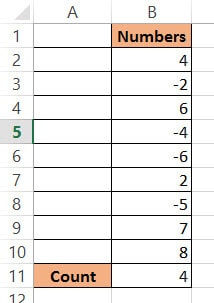## Method 1: Counting Negative Numbers using a Function

Excel’s COUNTIF function lets you count the number of cells in a range that match specified criteria. As such, this is the best way to count negative numbers in a range of cells.

Here’s the syntax for the COUNTIF function:

`= COUNTIF (range, condition)`

In this function,

• range is the range of cells containing the data you want the function to work on (or count)
• condition is the condition that you want to be satisfied in order to include a cell in the count.

The COUNTIF function goes through each cell in the range and counts only those cells that match the condition. It finally returns the total count of cells in the given range that match the criteria.

So if you want to count the number of negative numbers, the condition should be “<0”, because in order for a number to be considered as negative, it has to be less than 0.

Let us see how we can apply the COUNTIF function to count the negative numbers in our sample dataset:

1. Click on the cell where you want the result to be displayed. In our example, it will be cell B11.
2. Type the ‘equal to’ sign (=), followed by COUNTIF and an opening bracket: =COUNTIF(
3. Next, select the range of values that you want to count from. In our example, you have to select cells B2 to B10.
4. A reference to cells B2: B10 should appear after the opening bracket in cell B11.
5. Insert a comma (,) followed by the condition “<0”
6. Insert a closing bracket. Your complete formula in cell B11 for our example should be =COUNTIF(A2:A10, “<0”).
7. Press the Return key.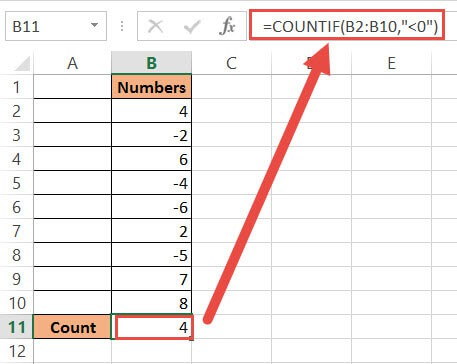You should now see the resulting count of negative numbers in cell B11.

In case you want to count all the cells that positive numbers in it, you can use the below formula:

`=COUNTIF(A2:A10, “>0”)`

## Method 2: Counting Negative Numbers using VBA

If you are alright with the idea of a little coding, then you can also use VBScript to programmatically get the count of negative numbers in a range.

Here’s the VBA code that you can use:

```Sub Count_negative_numbers()
Dim ws As Worksheet
Dim rng As Range
Dim result As Range
Set ws = Application.ActiveSheet
Set rng = Application.Selection
Set result = Application.InputBox( _
Title:="Get Location for Displaying Result", _
Prompt:="Select the cell where you want the result to appear", _
Type:=8)
result.Value = Application.WorksheetFunction.CountIf(rng, "<0")
End Sub```

This code takes a selection of cells and counts the number of cells containing negative numbers in that selection. It then asks the user to select the cell where they want the result to appear.

In case you want to count all the cells that have a positive number in it, simply change the CountIf(rng, “<0”) to CountIf(rng, “>0”) in the second last line in the code.

Once the user selects the cell, the code puts the result in the selected cell.

1. From the Developer Menu Ribbon, select Visual Basic.
2. Once your VBA window opens, Click Insert->Module. Copy the code given above and paste it into the module window.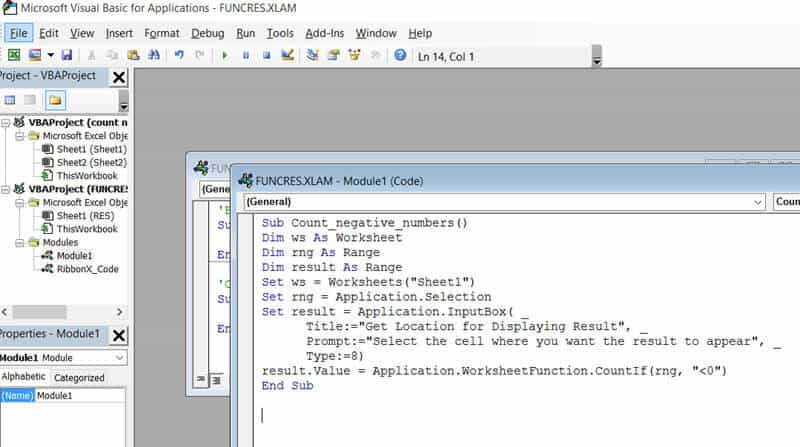3. Your code is now ready to run and use whenever needed. Close the VBA window.
4. Now go to your worksheet and select the range of cells containing the numeric values you want to work on (cells B2:B10 in our example).
5. Click on Macros, under the Developer tab.
6. This will open the Macros dialog box. Select the name Count_negative_numbers from the list of macros displayed.
7. Click OK.
8. Your code should now run. You will see a prompt asking you to select the cell where you want the result displayed. Select cell B11 for our example.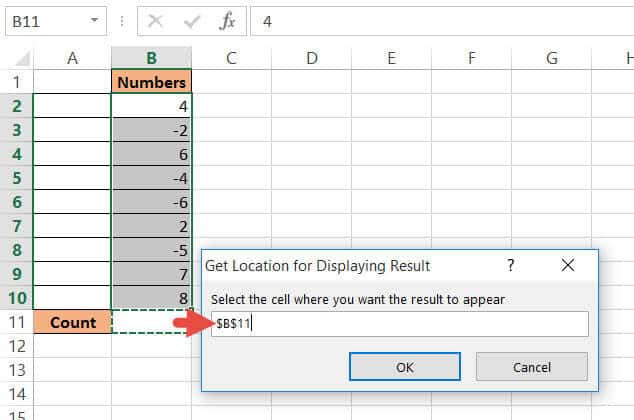9. You should see the result in your selected cell.Note that this method permanently changes the values in your worksheet. So there’s no way that you can undo it once the change is made.

If you need to use this macro multiple times, you can create a quick access button. Using this button, you can quickly count the negative numbers in a range of cells whenever you need to.

### Adding a Quick Access Button for the Macro

To add a button to quickly access the above macro, follow these steps:

1. Navigate to the File tab and then click on Options.
2. This will open the Excel Options dialog box. Select the Quick Access Toolbar option from the list on the left side of the dialog box.
3. Select Macros from the ‘Choose Commands From’ field.
4. Find the macro named Count_negative_numbers from the list of macros on the left.
5. Click the Add button. This will add the macro Count_negative_numbers to the Quick Access Toolbar. You should now see the macro’s name in the list on the right side of the dialog box.
6. Click OK to close the File Options dialog box.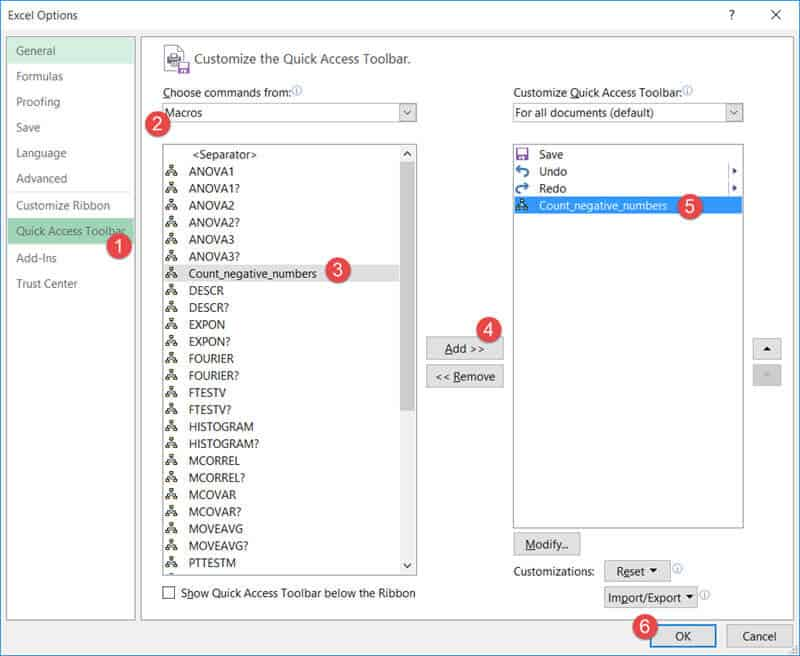7. You will now see the quick access button for your macro on the top of your Excel window.Whenever you select a range of number cells and click on this quick access button, your code will run and you can use it to count all the negative numbers in your selected range.

In this tutorial, we saw two easy ways to count negative numbers in a range of cells. The first method involves the use of the COUNTIF function with the condition “<0”. The second method involves the use of VBA code.

Note that you can select any range of numbers in any number of columns. The above two methods will help count the negative numbers in the entire selection. We hope this was helpful to you.

Other Excel tutorials you may like: